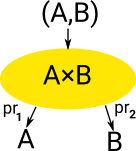# Maths - Product Types

 Product Type Has one constructor (formation) and multiple ways of deconstructing (elimination) (projections).### Notation

In type theory the product of A and B is denoted A×B. In logic the product is 'and' often denoted /\ .

An element of A×B is denoted (a,b) where 'a' is an element of 'A' and 'b' is an element of 'B'.

### Rules

Types are defined by their formation, term introduction, term eliminator and computation rules:

Introduction Elimination
 A B A/\B
(/\I)
 A/\B A
(/\E1)
 A/\B B
(/\E2)

This is discussed on the propositional logic page here.

 In type theory types are defined in terms of: Type formation Term introduction Term Elimination This picture shows how programming language constructs (In this case: Idris) relate to this theory:### β-reduction and η-reduction

#### β-reduction

construction followed by deconstruction:

• a = fst(a,b)
• b = snd(a,b)

#### η-reduction

deconstruction followed by construction:

• P = (fst P,snd P)

### Implementations in Idris

Product terms can be defined using 'data' but there is also a specific mechanism for products using 'record'.

 Here we start with a product type 'M'. We can deconstruct it by using 'fst' and 'snd' then construct it to get back to 'M' ```Idris> :let M = MkPair 2 "2" Idris> MkPair (fst M) (snd M) (2, "2") : (Integer, String) ``` Here we start with two terms 'x' and 'y' if we first construct using MkPair then deconstruct we get back to 'x' and 'y'. ```Idris> :let x = 3 Idris> :let y = "3" Idris> fst (MkPair x y) 3 : Integer Idris> snd (MkPair x y) "3" : String```

Other implementations of product

#### Idris

We can have a type which corresponds to conjunction:

 ```data And : Type -> Type -> Type where AndIntro : a -> b -> A a b```

from : Idris-dev/libs/prelude/Builtins.idr

```  ||| The non-dependent pair type, also known as conjunction.
||| @A the type of the left elements in the pair
||| @B the type of the right elements in the pair
data Pair : (A : Type) -> (B : Type) -> Type where
||| A pair of elements
||| @a the left element of the pair
||| @b the right element of the pair
MkPair : {A, B : Type} -> (1 a : A) -> (1 b : B) -> Pair A B```
from : Idris-dev/libs/prelude/ Prelude/Basics.idr
```||| Return the first element of a pair.
fst : (a, b) -> a
fst (x, y) = x

||| Return the second element of a pair.
snd : (a, b) -> b
snd (x, y) = y```

COQ

from : coq/theories/Init/Datatypes.v

```(** [prod A B], written [A * B], is the product of [A] and [B];
the pair [pair A B a b] of [a] and [b] is abbreviated [(a,b)] *)

#[universes(template)]
Inductive prod (A B:Type) : Type :=
pair : A -> B -> A * B

where "x * y" := (prod x y) : type_scope.

Add Printing Let prod.

Notation "( x , y , .. , z )" := (pair .. (pair x y) .. z) : core_scope.

Arguments pair {A B} _ _.

Register prod as core.prod.type.
Register pair as core.prod.intro.
Register prod_rect as core.prod.rect.

Section projections.
Context {A : Type} {B : Type}.

Definition fst (p:A * B) := match p with (x, y) => x end.
Definition snd (p:A * B) := match p with (x, y) => y end.

Register fst as core.prod.proj1.
Register snd as core.prod.proj2.
End projections.```

### Logic

In logic the product is 'and' denoted /\

## Relationship to Category Theory

Category theory is deeply related to type theory.

On this page is product is approached from the category theory direction and on this page is generalsed to the concept of a limit.

## Next Steps

Other types are discussed on the following pages:

Book Shop - Further reading.

Where I can, I have put links to Amazon for books that are relevant to the subject, click on the appropriate country flag to get more details of the book or to buy it from them.Computation and Reasoning - This book is about type theory. Although it is very technical it is aimed at computer scientists, so it has more discussion than a book aimed at pure mathematicians. It is especially useful for the coverage of dependant types.